# Area of parallelogram calculator

Area of parallelogram calculator - formulas and calculator for calculating area of parallelogram online. Formulas for calculating area of a parallelogram with various input data are given.

Parallelogram - is a geometric figure formed by four series-connected segments (sides), in which the opposite sides are parallel in pairs and equal to each other.

Area of parallelogram - is a numerical characteristic that characterizes the size of a plane bounded by a closed geometric figure formed by four series-connected segments (sides), in which the opposite sides are pairwise parallel and equal to each other.

Area - is a numerical characteristic characterizing the size of a plane bounded by a closed geometric figure.

Area is measured in units of measurement squared: km2, m2, cm2, mm2, etc.

1

## Parallelogram area by base and height of parallelogram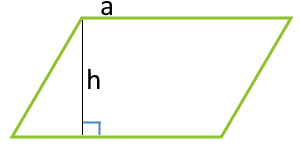a - side
h - height

... preparation ...

2

## Area of parallelogram along the side and the height lowered to this side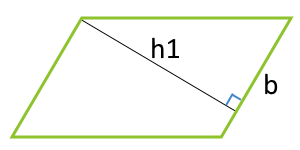b - side
hb - height

... preparation ...

3

## Area of parallelogram on two sides and the angle between them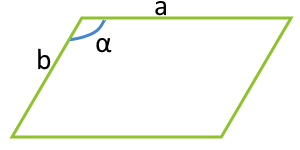a - side
b - side
α° - angle between parties

... preparation ...

4

## Area of parallelogram along the two diagonals and the angle between these diagonalsd1 - diagonal
d2 - diagonal
α° - Angle between diagonals

... preparation ...

5

## Area of parallelogram along the inscribed circle and the side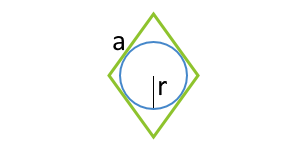This formula is applicable only for parallelograms, in which a circle can be inscribed. Such a parallelogram can only be a rhombus.

a - side
r - radius of inscribed circle

... preparation ...

6

## Area of parallelogram along the inscribed circle and the angle between the sides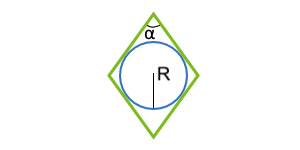This formula is applicable only for parallelograms, in which a circle can be inscribed. Such a parallelogram can only be a rhombus.

r - radius of inscribed circle
α° - angle between parties

... preparation ...